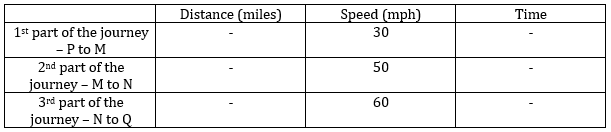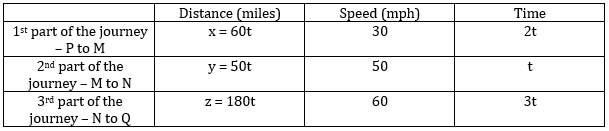Summer is Coming! Join the Game of Timers Competition to Win Epic Prizes. Registration is Open. Game starts Mon July 1st.

 It is currently 16 Jul 2019, 13:38### GMAT Club Daily Prep

#### Thank you for using the timer - this advanced tool can estimate your performance and suggest more practice questions. We have subscribed you to Daily Prep Questions via email.

Customized
for You

we will pick new questions that match your level based on your Timer History

Track

every week, we’ll send you an estimated GMAT score based on your performance

Practice
Pays

we will pick new questions that match your level based on your Timer History

#### Not interested in getting valuable practice questions and articles delivered to your email? No problem, unsubscribe here.# A straight highway connects two cities P and Q, and goes via two check

Author Message
TAGS:

### Hide Tags

e-GMAT RepresentativeV
Joined: 04 Jan 2015
Posts: 2942
A straight highway connects two cities P and Q, and goes via two check  [#permalink]

### Show Tags00:00

Difficulty:65% (hard)

Question Stats:57% (02:08) correct43% (02:35) wrongbased on 141 sessions

### HideShow timer StatisticsApplication of Average Speed in Distance problems - Exercise Question #1

A straight highway connects two cities P and Q, and goes via two checkpoints M and N, in that order. A bus started from P and moved to M at an average speed of 30 mph. After crossing M, it increased its speed to 50 mph and moved towards N. When it reached N, it further increased its speed to 60 mph and continued to move at this speed till it reached Q. What is the average speed of the bus throughout the whole journey?

Statement 1: The ratio of time the bus took to cover the distances PM, MN, and NQ respectively is 2:1:3
Statement 2: Out of the three distances, NQ is 3 times the distance PM and more than 3 times the distance MN

Option choices:
A) Statement (1) ALONE is sufficient, but statement (2) alone is not sufficient.
B) Statement (2) ALONE is sufficient, but statement (1) alone is not sufficient.
C) BOTH statements TOGETHER are sufficient, but NEITHER statement ALONE is sufficient.
D) EACH statement ALONE is sufficient.
E) Statements (1) and (2) TOGETHER are NOT sufficient.

To solve question 2: Question 2

To read the article: Application of Average Speed in Distance problems

_________________

Originally posted by EgmatQuantExpert on 09 May 2018, 08:31.
Last edited by EgmatQuantExpert on 13 Aug 2018, 06:12, edited 5 times in total.
e-GMAT RepresentativeV
Joined: 04 Jan 2015
Posts: 2942
Re: A straight highway connects two cities P and Q, and goes via two check  [#permalink]

### Show Tags

Solution

Given:
• A straight highway connects two cities P and Q, and goes via two checkpoints M and N, in that order
• A bus started from P and moved to M at an average speed of 30 mph
• After crossing M, it increased its speed to 50 mph, towards N
• From N, it further increased its speed to 60 mph till it reached Q

To find:
• The average speed of the bus throughout the whole journey

Approach and Working:
The given scenario can be depicted using the following table:Let us assume that the distance between P and M is x, between M and N is y, and between N and Q is z.
Therefore, the average speed of the whole journey = (x + y + z) / (x/30 + y/50 + z/60)

Analysing Statement 1
• As per the information given in Statement 1, the ratio of time the bus took to cover the distances PM, MN, and NQ respectively is 2:1:3
o Let us assume the time taken for the individual parts of the journey to be 2t, t, and 3t
• As we know the distances are x, y, and z respectively, we can say:
o $$\frac{x}{30}$$ = 2t or, x = 60t
o $$\frac{y}{50}$$ = t or, y = 50t
o $$\frac{z}{60}$$ = 3t or, z = 180t
• We can represent the present scenario as follows:• Therefore, the average speed = total distance travelled/ total time taken
o Or, average speed = (x + y + z)/(2t + t + 3t) = (60t + 50t + 180t)/6t = $$\frac{290t}{6t} = \frac{145}{3}$$ mph
• Hence, Statement 1 is sufficient to answer

Analysing Statement 2
• As per the information given in Statement 2, out of the three distances, NQ is 3 times the distance PM and more than 3 times the distance MN
o Given that distance NQ is more than 3 times the distance MN, we cannot get any exact value of MN
• Hence, Statement 2 is not sufficient to answer

Hence, the correct answer is Option A.

_________________
ManagerG
Joined: 28 Jul 2016
Posts: 132
Re: A straight highway connects two cities P and Q, and goes via two check  [#permalink]

### Show Tags

2
Math ExpertV
Joined: 02 Sep 2009
Posts: 56244
Re: A straight highway connects two cities P and Q, and goes via two check  [#permalink]

### Show Tags

LeonidK wrote:

____________________
Done. Thank you.
_________________Re: A straight highway connects two cities P and Q, and goes via two check   [#permalink] 10 Jul 2018, 14:01
Display posts from previous: Sort by

# A straight highway connects two cities P and Q, and goes via two check